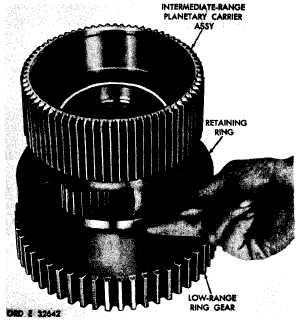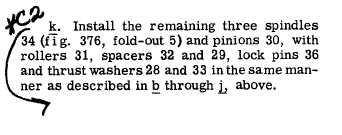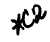Custom Search*C2 *C2 *C2 C H A P   5,   SEC   X X V I I N T E R M E D I A T E     P L A N E T A R Y ,     L O W     R I N G     G E A R     R E B U I LD PAR  191-195 the    planetary    carrier    and    position    the    spindle for    installation.    See    fig.    222.   Be   sure   that   the   slot   on   the spindle   is   indexed   properly   with   its lock   pin   bore   in   the   carrier. i With a press and spindle replacer 8351266,    install    planetary    carrier    spindle    (fig. 222).    The    spindle    replacer    will    bottom    against the   carrier   when   the   spindle   is   properly   posi- tioned   in   the   carrier. .   U s i n g    a    h a m m e r    a n d    p u n c h ,    i n s t a l l t h e    p l a n e t a r y    c a r r i e r    s p i n d l e    l o c k    p i n    ( f i g . 2 1 9 ) .    D r i v e    t h e    p i n    i n    0 . 0 3 0    t o    0 . 0 6 0    b e l o w the   surface   of   the   carrier.   Stake   metal   over the   pin. S e c t i o n    X X V I .    I N T E R M E D I A T E - R A N G E    P L A N E T A R Y    A N D    L O W - R A N G E   RING   GEAR   —   REBUILD 1 9 2 .    D E S C R I P T I O N Refer   to   pars.   12   and   13   for   the   descrip- tion    of    the    intermediate-range    planetary    and low-range    ring    gear. 1 9 3 .    D I S A S S E M B L Y    ( f i g .    3 7 6 ,    f o l d - o u t    5) .   All   related   items   not   covered in     through   ,   below,   were   removed from   the   transmission   as   outlined   in par.   75,   steps   116,   123   and   124.   No further   disassembly   of   these   parts   is r e q u i r e d . .   Remove   retaining   ring   that   retains   the i n t e r m e d i a t e - r a n g e    p l a n e t a r y    c a r r i e r    a s s e m- bly   in   the   low-range   ring   gear   (fig.   223).   Re- m o v e    t h e    c a r r i e r    a s s e m b l y . .   U s i n g    a    h a m m e r    a n d    p u n c h ,    r e m o ve four   planetary   carrier   spindle   lock   pins   (fig. 2 2 4 ) . .   Using   a   press   and   a   piece   of   3/8-inch drill   rod   approximately   6   inches   long,   or   other s u i t a b l e     p r e s s     t o o l ,     r e m o v e     f o u r     p l a n e t a ry spindles   from   the   carrier   (fig.   225). .    R e m o v e     p l a n e t a r y     c a r r i e r     p i n i o n s, spacers,    thrust    washers    and    bearing    rollers from   the   carrier   (fig.   225). 1 5 2 1 9 4 .     C L E A N I N G Refer   to   par.   71   for   cleaning   recommen- d a t i o n s. 1 9 5 .    I N S P E C T I O N    A N D    R E P A IR R e f e r    t o    p a r .    7 2    f o r    g e n e r a l    i n s p e c t i o n Figure  223.  Removing  (or  installing)  retaining  ring that retains intermediate-range planetary carrier assembly